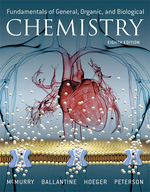×
Get Full Access to Fundamentals Of General, Organic, And Biological Chemistry (Mastering Chemistry) - 8 Edition - Chapter 22 - Problem 22.32
Get Full Access to Fundamentals Of General, Organic, And Biological Chemistry (Mastering Chemistry) - 8 Edition - Chapter 22 - Problem 22.32

×ISBN: 9780134015187 2044

## Solution for problem 22.32 Chapter 22

Fundamentals of General, Organic, and Biological Chemistry (Mastering Chemistry) | 8th Edition

• Textbook Solutions
• 2901 Step-by-step solutions solved by professors and subject experts
• Get 24/7 help from StudySoup virtual teaching assistantsFundamentals of General, Organic, and Biological Chemistry (Mastering Chemistry) | 8th Edition

4 5 1 272 Reviews
12
5
Problem 22.32

Complete the following word equation:

Lactose + $$H_{2}O \rightarrow ? + ?$$

Where in the digestive system does this process occur?

Text Transcription:

H_2O? + ?

Step-by-Step Solution:
Step 1 of 3

Chapter6  Energy: The scientific description of changes that take place in the physical world is centered around the concept of energy. The concept of energy is deeply entangled with the idea of objects in motion. Objects in motion are said to have kinetic energy. Stored energy that is capable of causing an object to move in the future is called potential energy. One of the fundamental postulates of science is the Law of Conservation of Energy. It states that energy is neither created nor destroyed in any physical process – it is just converted from one form to another or moved from place to place. The total energy of the universe remains constant. The Law of Conservation of Energy is also known as the First Law of Thermodynamics. The various forms of energy are classified in many ways, depending upon the identity of the objects and the forces acting upon them, the direction of the motion, and whether or not the energy is kinetic or potential. Forms of Energy Thermal energy Translational energy Nuclear energy Rotational energy Electrical energy Vibrational energy Mechanical energy Chemical energy Virtually all forms of energy can be classified as kinetic energy or potential energy. Kinetic energy is a well-defined quantity that is a function of the mass (m) of the moving object and its speed (v) relative to a fixed point. Kinetic Energy = ½ m v2 The basic S.I. unit for all forms of energy is the joule. 1 joule (J) = 1 kg m2 s-2 Note that these units come directly fr

Step 2 of 3

Step 3 of 3

##### ISBN: 9780134015187

The answer to “?Complete the following word equation:Lactose + $$H_{2}O \rightarrow ? + ?$$Where in the digestive system does this process occur?Text Transcription:H_2O ? + ?” is broken down into a number of easy to follow steps, and 23 words. This full solution covers the following key subjects: . This expansive textbook survival guide covers 281 chapters, and 240 solutions. This textbook survival guide was created for the textbook: Fundamentals of General, Organic, and Biological Chemistry (Mastering Chemistry), edition: 8. Since the solution to 22.32 from 22 chapter was answered, more than 202 students have viewed the full step-by-step answer. The full step-by-step solution to problem: 22.32 from chapter: 22 was answered by Aimee Notetaker, our top Chemistry solution expert on 04/25/22, 03:57PM. Fundamentals of General, Organic, and Biological Chemistry (Mastering Chemistry) was written by Aimee Notetaker and is associated to the ISBN: 9780134015187.

Unlock Textbook Solution